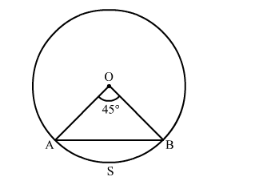# In Fig. 15.76, O is the centre of circle of radius 28 cm.Question:

In Fig. 15.76, O is the centre of circle of radius 28 cm. Find the area of minor segment ASB.Solution:

We have given that radius of the circle is $28 \mathrm{~cm}$ and sector angle is $45^{\circ}$.

Now we will find the area of the minor segment ASB.

$\therefore$ Area of the minor segment $A S B=$ Area of the sector $O A S B$ - Area of the $\triangle O A B$

$\therefore$ Area of the minor segment $\mathrm{ASB}=\frac{\theta}{360} \times \pi r^{2}-\frac{1}{2} \times O A \times O B$

$\therefore$ Area of the minor segment $A S B=\frac{45}{360} \times 22 \times 4 \times 28-14 \times 28 \times \frac{1}{\sqrt{2}}$

$\therefore$ Area of the minor segment $\mathrm{ASB}=\frac{45}{360} \times \frac{22}{7} \times 28 \times 28-\frac{1}{2} \times 28 \times 28 \times \sin 45$

$\therefore$ Area of the minor segment $\mathrm{ASB}=\frac{45}{360} \times 22 \times 4 \times 28-14 \times 28 \times \frac{1}{\sqrt{2}}$

$\therefore$ Area of the minor segment $A S B=\frac{1}{8} \times 22 \times 4 \times 28-14 \times 28 \times \frac{1}{\sqrt{2}}$

$\therefore$ Area of the minor segment $\mathrm{ASB}=\frac{1}{8} \times 22 \times 4 \times 28-14 \times 28 \times \frac{1}{\sqrt{2}}$

$\therefore$ Area of the minor segment $\mathrm{ASB}=11 \times 28-14 \times 28 \times \frac{1}{\sqrt{2}}$

$\therefore$ Area of the minor segment $\mathrm{ASB}=308-392 \times \frac{1}{\sqrt{2}}$

$\therefore$ Area of the minor segment $\mathrm{ASB}=308-\sqrt{2} \times 196$

$\therefore$ Area of the minor segment ASB $=308-1.414 \times 196$

$\therefore$ Area of the minor segment $\mathrm{ASB}=30.856$

Therefore, area of the minor segment ASB is $30.856 \mathrm{~cm}^{2}$